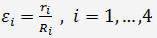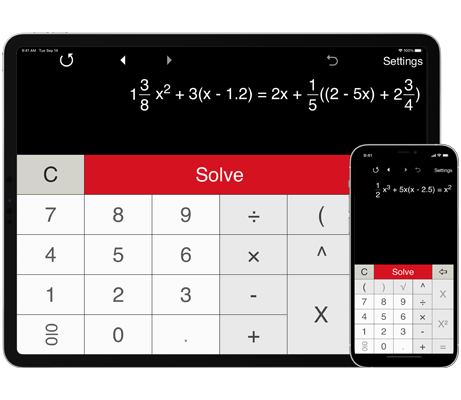# Quartic Equation Calculator

Quartic Equation Calculator solves a wide range of quartic, cubic, quadratic and linear equations, including equations with fractions and parentheses.
With the Advanced format, you can input equations in any form.

## How to solve a quartic equation using Quartic Equation Calculator

Quartic Equation Calculator solves quartic, cubic, quadratic and linear equations, including equations with fractions and parentheses. Just type in any equation you want to solve and Quartic Equation Calculator will show you the result.

Quartic Equation Calculator supports the predefined format (in the Settings window) for quartic equations (or fourth degree equations) in the general form:
ax4 + bx3 + cx2 + dx + e = 0.
To solve a fourth degree equation, enter the coefficients 'a', 'b', 'c', 'd' and 'e' and press 'Solve'. The coefficients 'a', 'b', 'c', 'd' and 'e' are real numbers, a ≠ 0. Note: for a missing term enter zero.

Quartic Equation Calculator displays the original equation and the result.

Quartic Equation Calculator supports positive, negative, or zero values of the coefficients.

#### Solving a fourth degree equation (quartic equation)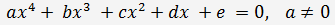(1)

1. Using the substitution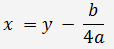we get the depressed equation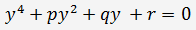(2),   where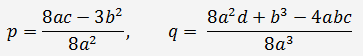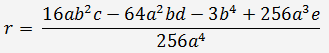2. If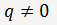, we will solve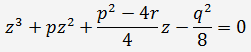If, then this equation always has a positive root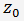The roots of the original quartic equation (1) can be obtained by the formulas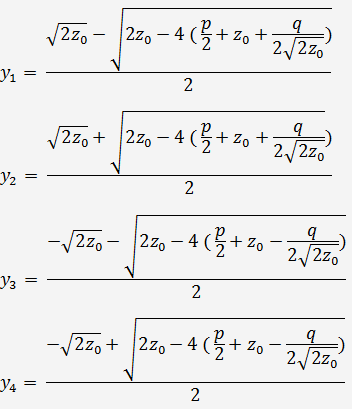3. If q = 0, then the reduced equation (2) becomes a biquadratic equation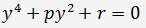And the four roots of this equation can be found by the formulas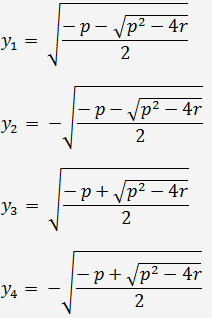4. You can estimate the solutions with the corresponding discrepancies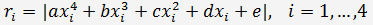The less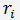is, the more accurate the solutions are. For a more accurate estimate is reasonable to consider the relative error.

Solve the equation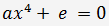and calculate the corresponding discrepancies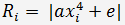Then the relative discrepancies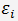can be calculated